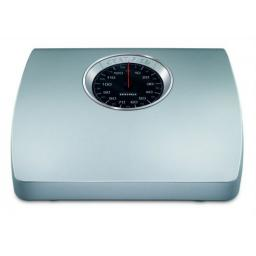# Determine 3033

Andrej, Michal, Patrik, and Juraj together weigh 128 kg. Their weights are in the ratio 1:6:7:2. Determine what weight each of them has.

a =  8
j =  16
m =  48
p =  56

### Step-by-step explanation:

a+m+p+j=128
m = 6a
p = 7a
j=2a

a+m+p+j=128
m = 6·a
p = 7·a
j=2·a

a+j+m+p = 128
6a-m = 0
7a-p = 0
2a-j = 0

Pivot: Row 1 ↔ Row 3
7a-p = 0
6a-m = 0
a+j+m+p = 128
2a-j = 0

Row 2 - 6/7 · Row 1 → Row 2
7a-p = 0
-m+0.8571p = 0
a+j+m+p = 128
2a-j = 0

Row 3 - 1/7 · Row 1 → Row 3
7a-p = 0
-m+0.8571p = 0
j+m+1.1429p = 128
2a-j = 0

Row 4 - 2/7 · Row 1 → Row 4
7a-p = 0
-m+0.8571p = 0
j+m+1.1429p = 128
-j+0.2857p = 0

Pivot: Row 2 ↔ Row 3
7a-p = 0
j+m+1.1429p = 128
-m+0.8571p = 0
-j+0.2857p = 0

Row 4 + Row 2 → Row 4
7a-p = 0
j+m+1.1429p = 128
-m+0.8571p = 0
m+1.4286p = 128

Row 4 + Row 3 → Row 4
7a-p = 0
j+m+1.1429p = 128
-m+0.8571p = 0
2.2857p = 128

p = 128/2.28571429 = 56
m = 0-0.85714285714286p/-1 = 0-0.85714286 · 56/-1 = 48
j = 128-m-1.1428571428571p = 128-48-1.14285714 · 56 = 16
a = 0+p/7 = 0+56/7 = 8

a = 8
j = 16
m = 48
p = 56

Our linear equations calculator calculates it.Did you find an error or inaccuracy? Feel free to write us. Thank you!

Tips for related online calculators
Check out our ratio calculator.
Do you have a linear equation or system of equations and looking for its solution? Or do you have a quadratic equation?